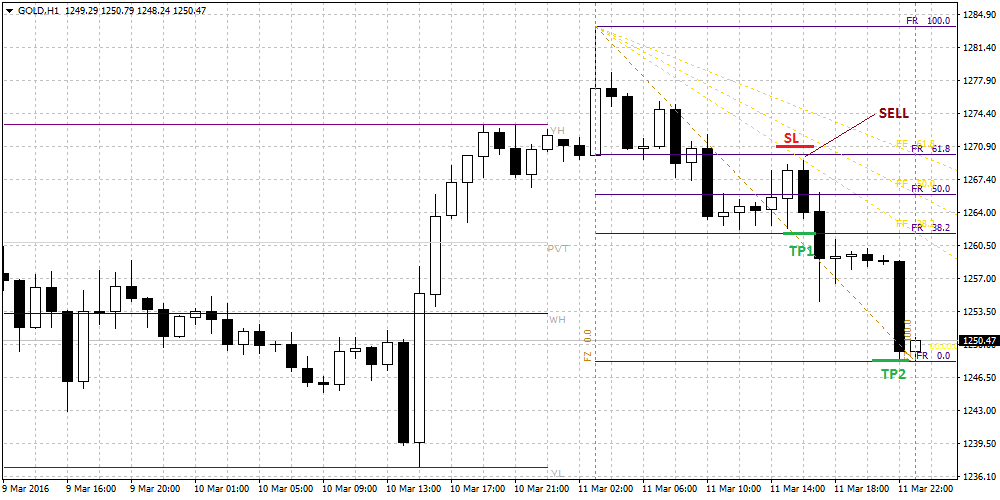# Price Retracements Fibonacci Forex StrategyThe forex trading strategy discussed here is the use of the iFibonacci.ex4 forex indicator to trade price retracements.

This indicator is based on the Fibonacci numbers and can be used to create several strategies around these Fibonacci numbers.

One of these strategies is the retracement strategy.

Chart Setup

MetaTrader4 Indicators: iFibonacci.ex4 (default setting)

Preferred Time Frame(s):  30-Minutes, 1-Hour, 4-Hours,

Recommended Trading Sessions: Any time the signal appears on the charts.

Currency Pairs: Any + Metals & Oil

Download The Price Retracements Fibonacci Forex Strategy

Buy Trade Example (click the image for full size)STRATEGY

When the iFibonacci.ex4 indicator is placed on the charts, the Fibo fans and retracement lines appear. The colour of the retracement lines are changed to make them more visible and distinct from the fan lines. This can be done using Ctrl + I, then clicking the iFibonacci.ex4 tab and then clicking “Edit”. Scroll down to the retracement component of the indicator, and change the colour under the “Colour” tab.

Long Entry Rules:

A long position is initiated when the following indicator pattern is displayed on the chart:

• Price action retraces to a Fibonacci retracement line. The price candle must bounce off this line i.e. must touch the retracement line without closing below the line. This is shown at the 61.8% retracement line in this example.
• Once the candle has touched the line without closing below it, set a long entry at the open of the next candle. If the previous trigger candle closes at some distance from the fan line, allow the price to pull back before initiating the long entry.

Stop Loss for Long Entry

A few pips below the Fibo retracement line where the long trade is initiated.

Exit Strategy/Take Profit for Long Entry:

• For this indicator, you can use the retracement line immediately above the entry line. If price action breaks this line, use the next retracement level above this. In the snapshot, the entry was made at the 61.8% line. The price broke above the 50% line, so the next TP level is at the 38.2% line.

Short Entry Rules:

For the short entry, the trader should look for the following:

• Price is rejected off a Fibonacci retracement line. In this example, this occurs at the 61.8% line.
• The short entry should be made as shown on the chart below.Stop Loss for Sell Entry

A few pips above the Fibo retracement line where trade entry is made.

Exit Strategy/Take Profit for Sell Entry

To take profit from this trade, the following should be used.

• The Fibo retracement line located immediately below the trade entry point. If the price action is so strong as to break this landmark, then the Fibo retracement below should be considered. In this example, trade entry was at 61.8% line. Price broke the 50% line, so the next TP is set at the 38.2% line. Price still broke this level, and eventually went all the way to the 0.00% line.